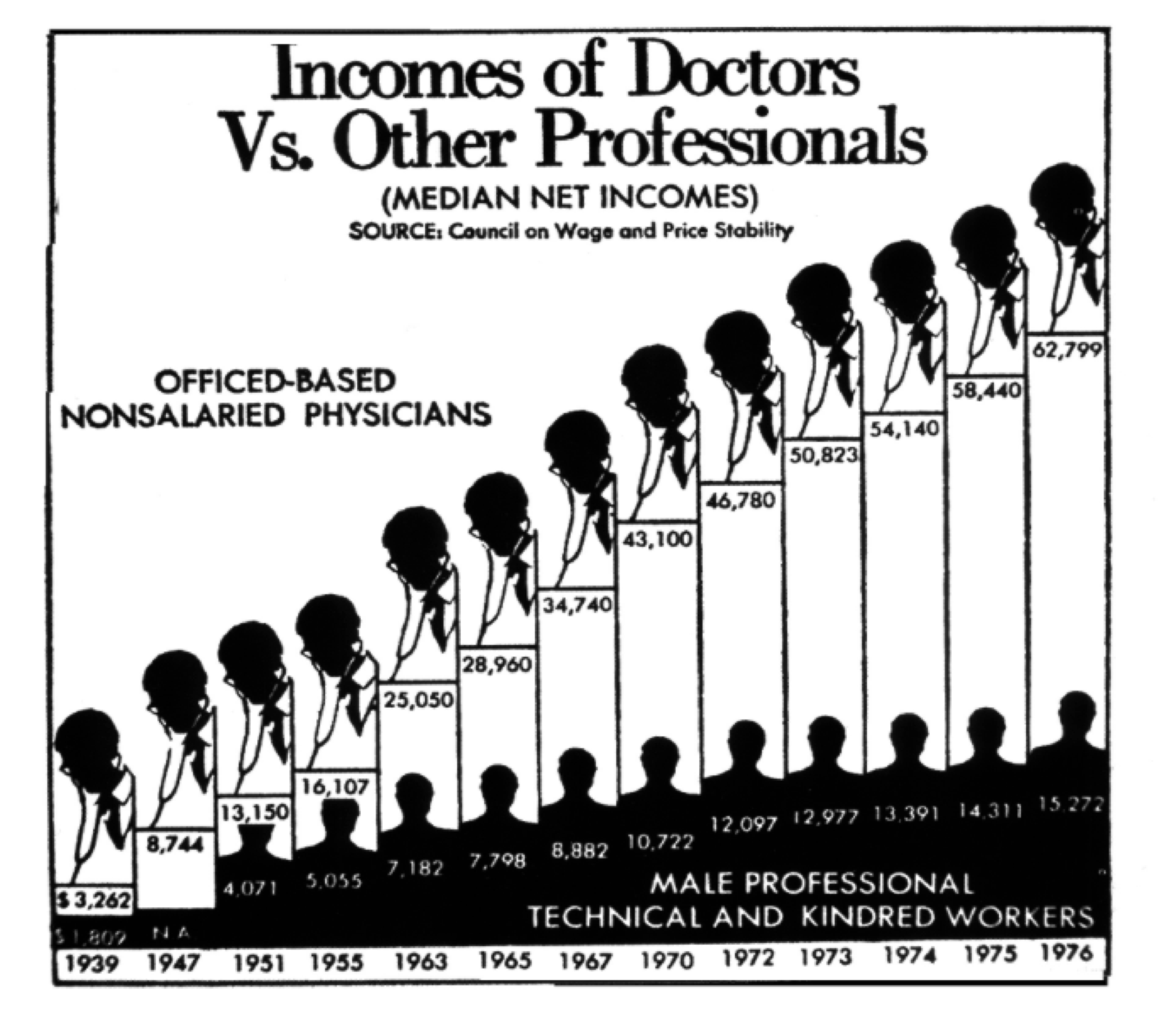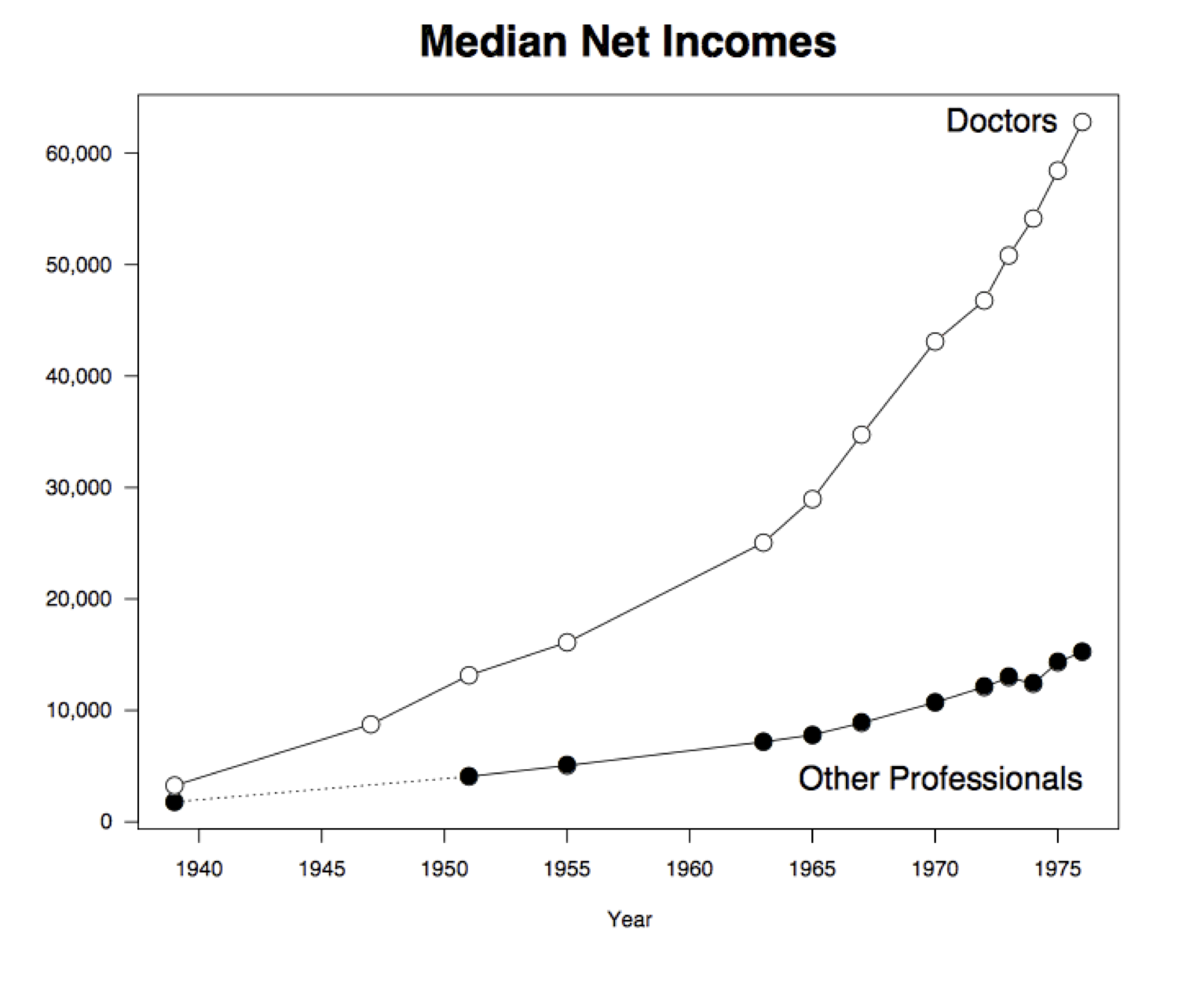# Expressions

Programming languages are much simpler than human languages. Nonetheless, there are some rules of grammar to learn in any language, and that is where we will begin. In this text, we will use the Python programming language. Learning the grammar rules is essential, and the same rules used in the most basic programs are also central to more sophisticated programs.

Programs are made up of expressions, which describe to the computer how to combine pieces of data. For example, a multiplication expression consists of a * symbol between two numerical expressions. Expressions, such as 3 * 4, are evaluated by the computer. The value (the result of evaluation) of the last expression in each cell, 12 in this case, is displayed below the cell.

3 * 4

12

The grammar rules of a programming language are rigid. In Python, the * symbol cannot appear twice in a row. The computer will not try to interpret an expression that differs from its prescribed expression structures. Instead, it will show a SyntaxError error. The Syntax of a language is its set of grammar rules, and a SyntaxError indicates that an expression structure doesn't match any of the rules of the language.

3 * * 4

  File "<ipython-input-4-d90564f70db7>", line 1
3 * * 4
^
SyntaxError: invalid syntax


Small changes to an expression can change its meaning entirely. Below, the space between the *'s has been removed. Because ** appears between two numerical expressions, the expression is a well-formed exponentiation expression (the first number raised to the power of the second: 3 times 3 times 3 times 3). The symbols * and ** are called operators, and the values they combine are called operands.

3 ** 4

81

Common Operators. Data science often involves combining numerical values, and the set of operators in a programming language are designed to so that expressions can be used to express any sort of arithmetic. In Python, the following operators are essential.

Expression Type Operator Example Value
Addition + 2 + 3 5
Subtraction - 2 - 3 -1
Multiplication * 2 * 3 6
Division / 7 / 3 2.66667
Remainder % 7 % 3 1
Exponentiation ** 2 ** 0.5 1.41421

Python expressions obey the same familiar rules of precedence as in algebra: multiplication and division occur before addition and subtraction. Parentheses can be used to group together smaller expressions within a larger expression.

1 + 2 * 3 * 4 * 5 / 6 ** 3 + 7 + 8 - 9 + 10

17.555555555555557
1 + 2 * (3 * 4 * 5 / 6) ** 3 + 7 + 8 - 9 + 10

2017.0

### Example¶

Here, from the Washington Post in the early 1980s, is a graph that attempts to compare the earnings of doctors with the earnings of other professionals over a few decades. Do we really need to see two heads (one with a stethoscope) on each bar? Edward Tufte, Professor at Yale and one of the world's experts on visualizing quantitative information, coined the term "chartjunk" for such unnecessary embellishments. This graph is also an example of the "low data-to-ink ratio" that Tufte deplores.Most importantly, the horizontal axis of the graph is is not drawn to scale. This has a significant effect on the shape of the bar graphs. When drawn to scale and shorn of decoration, the graphs reveal trends that are quite different from the apparently linear growth in the original. The elegant graph below is due to Ross Ihaka, one of the originators of the statistical system R.In the period 1939 to 1963, the doctors' incomes went up from \$3,262 to \$25,050. So during that period the average increase in income per year was about \$900. (25050 - 3262)/(1963 - 1939)  907.8333333333334 In Ross Ihaka's graph you can see that in this period, the doctors' incomes rise roughly linearly at a fairly steady rate. That rate is about \$900, as we have just calculated.

But in the period 1963 to 1976, the rate is more than three times as high:

(62799 - 25050)/(1976 - 1963)

2903.769230769231

That is why the graph rises much more steeply after 1963.

This chapter introduces many types of expressions. Learning to program involves trying out everything you learn in combination, investigating the behavior of the computer. What happens if you divide by zero? What happens if you divide twice in a row? You don't always need to ask an expert (or the Internet); many of these details can be discovered by trying them out yourself.